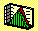2.8 SAMPLE STATISTICS: SUFFICIENCY

This statistical concept of sufficiency approaches the property of a statistic from an informational perspective. It can be motivated by asking whether an estimate of some underlying population parameter using a sample statistic can be improved upon by using both the sample and a statistic constructed from the sample. In finance, the concept of sufficiency is used in modeling the strong form of the efficient market hypothesis, that price fully reflects all information about a security. For our purposes here, the question addressed is whether an investor's preference-maximizing demand for some risky security, when based upon both price and the investor's private information, is different from the preference-maximizing demand based upon price alone? If the security price is a sufficient statistic, then the answer is no.

Next, we apply the statistical concepts developed in Chapter 2 to portfolios of securities.

previous topic

next topic